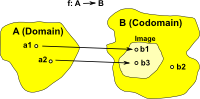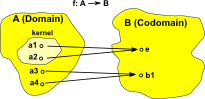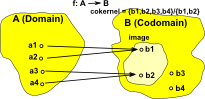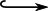# Maths - Maps

## Functions (mappings)

A function (or mapping) φ from a set 'A' to a set 'B' is a rule that assigns to each element 'a' of A' exactly one element 'b' of B. This is written:

φ: A -> B

## Domain and Codomain

 Domain This is the set which is the source of the function.Codomain This is the set which is the destination of the function.## Total Functions

Function which give an output for every possible value of the domain.

See injective function below.

## Partial Functions

On this page we will mostly assume that every element in the domain maps to an element in the domain, however this is not the case for partial functions.

Examples of partial functions, in the real numbers, are:

• x->1/x (not defined for x=0)
• x->sqrt(x) (not defined for negative numbers)

This is also interesting in computing where a function may represent a computation. Where this computation is very complicated it may not be possible to be sure that every input to the function will produce a valid result.

The mathematics of partial functions is discussed further on the page here.

## Injective, Surjective and Bijective Functions

 Injective function Every element in the domain is mapped to a unique element in the codomain. There may be elements in the domain that are not used. (size of domain <= size of codomain)Surjective (onto) Elements in the domain may be mapped to the same element in the codomain but every element in the codomain is targeted. (size of domain >= size of codomain)Bijective function (one-to-one correspondence) (Injective and Surjective) Every element in the domain is mapped to a unique element in the codomain and every element in the codomain is mapped to a unique element in the domain. (size of domain = size of codomain)## Ways to Define a Function

• By specifying an algorithm.
• By specifying a set of ordered pairs. (In ZF set theory everything is a set). Either by specifying each pair or by a comprehension.

An important feature and a definition of functions is that a given input produces a unique output:

if <x,y>f and <x,z>f then y=z

## Ways to Define Equality of Functions

The (ZF) set theory way to define a relation is as a set of ordered pairs.

## Composition of Functions

If we apply a function 'g' to and element x and then apply function h' to the result we get a combined function: (g o h)(x)

where:

• o represents the composition of these two functions.

In other words we have:

(g o h)(x) = h(g(x))Function composition is always associative

(f o g)o h = f o (g o h)

## MorphismsA morphism is a function f between sets with structure (such as group, ring or fields) that preserve some element of the structure.

For instance :

f(g * h) = f(g) * f(h)

### Types of Morphisms

 morphism homomorphism general form, same meaning as homomorphism. isomorphism a morphism where the inverse f-1 is also a morphism. f-1(g * h) = f-1(g) * f-1(h) automorphism a isomorphism onto itself endomorphism a morphism onto itself

#### Examples

Morphisms between Lattices on page here.

## Image and Kernel

 ImageKernel The set of all elements 'x' of a structure 'X' such that f(x) is the identity element of structure Y.Coimage the coimage of a morphism f: A -> B is the quotient: coim f = A/ker(f)Cokernel the cokernel of a linear mapping of vector spaces f : A -> B is the quotient: cokernel = B/im(f)## Orbit and Stabiliser

see Sylow theory

### Orbit

orb(s) - When a group G acts on a set S, the orbit of any s in S is the set of elements of S that G arrows can reach from s.

orb(s) = {φ(s) | φG}### Stabiliser

stab(s) - The stabilizer of an element s in S is the set of group elements g that don't move s. A configuration s in S is called stable if no actions move s.

stab(s) = {φG | φ(s)=s}### Types of Map

notation example meaning
AB   Map / Morphism / Function between mathematical structures that relates or preserves the structures in some way. May be injection, surjection or bijection (injection can be specifically indicated see below)
ab   A function in terms of elements of the structures aA, bBA function that is valid if all the functions drawn as solid lines are validinjective morphism (embedding)canonical map - map of G onto factor group G/H where H is a normal subgroup of G

 metadata block see also: Correspondence about this page Book Shop - Further reading. Where I can, I have put links to Amazon for books that are relevant to the subject, click on the appropriate country flag to get more details of the book or to buy it from them.Mathematics for 3D game Programming - Includes introduction to Vectors, Matrices, Transforms and Trigonometry. (But no euler angles or quaternions). Also includes ray tracing and some linear & rotational physics also collision detection (but not collision response). Other Math Books Specific to this page here: